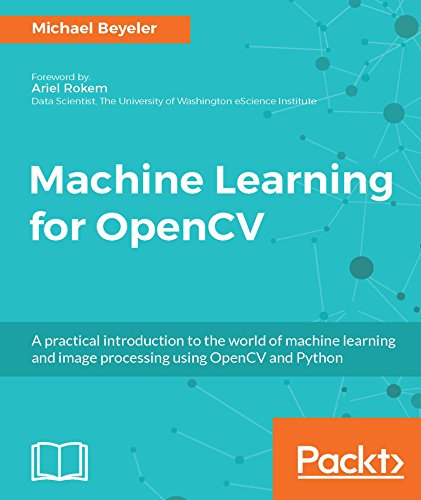# EnzoLiang / opencv-machine-learning

Machine Learning for OpenCV: A practical introduction to the world of machine learning using OpenCV and Python

Geek Repo

Github PK Tool

# Machine Learning for OpenCV

This is the Jupyter notebook version of the following book:Michael Beyeler
Machine Learning for OpenCV: A practical introduction to the world of machine learning and image processing using OpenCV and Python

14 July 2017
Packt Publishing Ltd., London, England
Paperback: 382 pages
ISBN 978-178398028-4

The content is available on GitHub. The code is released under the MIT license.

For questions, discussions, and more detailed help please refer to the Google group.

If you use either book or code in a scholarly publication, please cite as:

M. Beyeler, (2017). Machine Learning for OpenCV. Packt Publishing Ltd., London, England, 380 pages, ISBN 978-178398028-4.

Or use the following bibtex:

``````@book{MachineLearningOpenCV,
title = {{Machine Learning for OpenCV}},
subtitle = {{A practical introduction to the world of machine learning and image processing using OpenCV and Python}},
author = {Michael Beyeler},
year = {2017},
pages = {380},
publisher = {Packt Publishing Ltd.},
isbn = {978-178398028-4}
}
``````

Preface

Foreword by Ariel Rokem

## Running the Code

There are at least two ways you can run the code:

• Using Binder (no installation required).
• Using Jupyter Notebook on your local machine.

The code in this book was tested with Python 3.5, although older versions of Python should work as well (such as Python 2.7).

### Using Binder

Binder allows you to run Jupyter notebooks in an interactive Docker container. No installation required!

Launch the project: mbeyeler/opencv-machine-learning

### Using Jupyter Notebook

You basically want to follow the installation instructions in Chapter 1 of the book.

In short:

1. Download and install Python Anaconda. On Unix, when asked if the Anaconda path should be added to your `PATH` variable, choose yes. Then either open a new terminal or run `\$ source ~/.bashrc`.

2. Fork and clone the GitHub repo:

• Click the `Fork` button in the top-right corner of this page.
• Clone the repo, where `YourUsername` is your actual GitHub user name:
``````\$ git clone https://github.com/YourUsername/opencv-machine-learning
\$ cd opencv-machine-learning
``````
``````\$ git remote add upstream https://github.com/mbeyeler/opencv-machine-learning
``````
3. Add Conda-Forge to your trusted channels (to simplify installation of OpenCV on Windows platforms):

``````\$ conda config --add channels conda-forge
``````
4. Create a conda environment for Python 3 with all required packages:

``````\$ conda create -n Python3 python=3.5 --file requirements.txt
``````
5. Activate the conda environment. On Linux / Mac OS X:

``````\$ source activate Python3
``````

On Windows:

``````\$ activate Python3
``````

6. Launch Jupyter notebook:

``````\$ jupyter notebook
``````

This will open up a browser window in your current directory. Navigate to the folder `opencv-machine-learning`. The README file has a table of contents. Else navigate to the `notebooks` folder, click on the notebook of your choice, and select `Kernel > Restart & Run All` from the top menu.

## Getting the latest code

If you followed the instructions above and:

• forked the repo,
• cloned the repo,
• added the `upstream` remote repository,

then you can always grab the latest changes by running a git pull:

``````\$ cd opencv-machine-learning
\$ git pull upstream master
``````

## Errata

The following errata have been reported that apply to the print version of the book:

• p.32: `Out` should read '3' instead of 'int_arr'.
• p.32: `Out` should read `array([9, 8, 7, 6, 5, 4, 3, 2, 1, 0])` instead of `array([0, 1, 2, 3, 4, 5, 6, 7, 8, 9])`.
• p.72: `In ` should read `ridgereg = linear_model.Ridge()` instead of `ridgereg = linear_model.RidgeRegression()`.
• p.120: `In ` should read `dtree = cv2.ml.DTrees_create()` instead of `dtree = cv2.ml.dtree_create()`.
• p.122: `In ` should read `with open("tree.dot", 'w'): f = tree.export_graphviz(dtc, out_file=f, feature_names=vec.get_feature_names(), class_names=['A', 'B', 'C', 'D'])` instead of `with open("tree.dot", 'w'): f = tree.export_graphviz(clf, out_file=f)`.
• p.147: The first occurrences of `X_hypo = np.c_[xx.ravel().astype(np.float32), yy.ravel().astype(np.float32)]` and `_, zz = svm.predict(X_hypo)` should be removed, as they mistakenly appear twice.
• p.193: `In ` is missing `from sklearn import metrics`.
• p.201: Indentation in bullet points 2-4 are wrong. Please refer to the Jupyter notebook for the correct indentation.
• p.230: `In ` has wrong indentation: `class Perceptron(object)` correctly has indentation level 1, but `def __init__` should have indentation level 2, and the two commands `self.lr = lr; self.n_iter = n_iter` should have indentation level 3.
• p.260: `In ` should read `from keras.models import Sequential` instead of `from keras.model import Sequential`.
• p.260: `In ` should read `model.add(Conv2D(n_filters, (kernel_size, kernel_size), padding='valid', input_shape=input_shape))` instead of `model.add(Convolution2D(n_filters, kernel_size, kernel_size, border_mode='valid', input_shape=input_shape))`.
• p.260: `In ` should read `model.add(Conv2D(n_filters, (kernel_size, kernel_size)))` instead of `model.add(Convolution2D(n_filters, (kernel_size, kernel_size)))`.
• p.261: `In ` should read `model.fit(X_train, Y_train, batch_size=128, epochs=12, verbose=1, validation_data=(X_test, Y_test))` instead of `model.fit(X_train, Y_train, batch_size=128, nb_epoch=12, verbose=1, validation_data=(X_test, Y_test))`.
• p.275, in bullet point 2 it should say `ret = classifier.predict(X_hypo)` instead of `zz = classifier.predict(X_hypo); zz = zz.reshape(xx.shape)`.
• p.285: `plt.imshow(X[i, :].reshape((64, 64)), cmap='gray')` should be indented so that it is aligned with the previous line.
• p.288: `In ` should read `_, y_hat = rtree.predict(X_test)` instead of `_, y_hat = tree.predict(X_test)`.
• p.306: `In ` should read `from sklearn.model_selection import train_test_split` instead of `from sklearn.model_selection import model_selection`.
• p.310: `In ` should read `knn.train(X_boot, cv2.ml.ROW_SAMPLE, y_boot)` instead of `knn.train(X_train, cv2.ml.ROW_SAMPLE, y_boot)`.
• p.328: `In ` is missing the statement `from sklearn.preprocessing import MinMaxScaler`.

Please note that these mistakes do not appear in the code of this repository.

## Acknowledgment

This book was inspired in many ways by the following authors and their corresponding publications:

These books all come with their own open-source code - check them out when you get a chance!